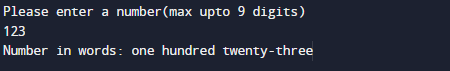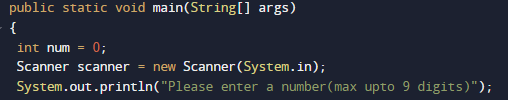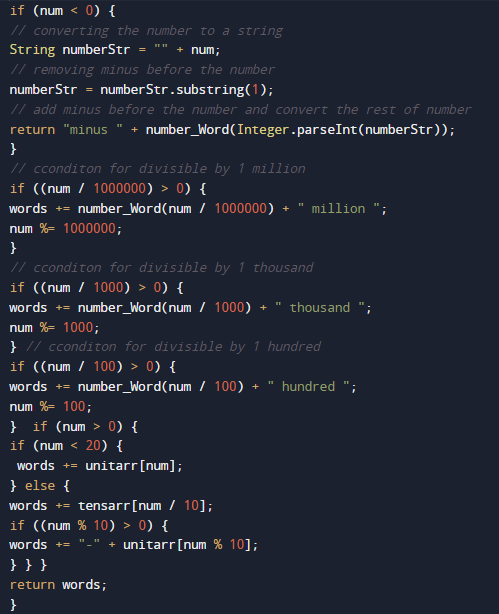# Java Program to convert Number to Word

In this tutorial you will learn about the Java Program to convert Number to Word and its application with practical example.

In this tutorial, we will learn to create a Java  Program to convert Number to Word.

## Prerequisites

Before starting with this tutorial we assume that you are best aware of the following java programming topics:

• Java Operators.
• Basic Input and Output
• Class and Object.
• Basic Java programming.
• String.
• User define Function.

## Java Program to convert Number to Word

In this program we will convert Number to Word. We would first declared and initialized the required variables. Next, we would ask user to enter a number. let have a look at Code.

## OutputIn the above program, we have first declared and initialized a set variables required in the program.

• num = it will hold entered Number
• word= it will hold word values.
• unitarr[]= it will hold unit value of word.
• tensarr[]=it will hold tense value of word.

After that we declare variables and taking value from user.the following  code supports numbers up-to 9999 digits, i.e., numbers from 0 to 9999.

here we create arrays that break individual parts of a strings. One array is used for single digits (unitarr[]), one for numbers from 10 to 19,. etc (tensarr[]). and one for powers of 10. and multiple condition that number is >1000 ,>100 ans so on..as shoen in image below.And finally we will print the characters or given numbers.In this tutorial we have learn about the Java Program to convert Number to Word and its application with practical example. I hope you will like this tutorial.